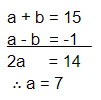# The sum of the digits of a two-digit number is 15.

Question:

The sum of the digits of a two-digit number is 15. The number obtained by interchanging its digits exceeds the given number by 9. Find the original number.

Solution:

Let the tens place digit be a and the units place digit be b.

Then, the number is (10a + b).

​Given:

a + b = 15         ... (1)

When the digits are interchanged the number will be (10 b + a).

Given:

10a + b + 9 = 10 b + a

∴ 10a - a + b - 10b = -9

9a - 9b = -9​

a - b = -1            ... (2)Original number $=10 a+b=10 \times 7+8=78$